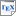[Gir14] Sergio Giro. Optimal Schedulers vs Optimal Bases: An approach for efficient exact solving of Markov decision processes. Theoretical Computer Science, 538, pages 70–83, Elsevier. June 2014. [pdf] [bib] [Investigates exact-arithmetic model checking methods for MDPs, implemented in an extension of PRISM.] Downloads:pdf (541 KB)bib Links: [Google] [Google Scholar] [CiteSeer] Abstract. Quantitative model checkers for Markov Decision Processes typically use finite-precision arithmetic. If all the coefficients in the process are rational numbers, then the model checking results are rational, and so they can be computed exactly. However, exact techniques are generally too expensive or limited in scalability. In this paper we propose a method for obtaining exact results starting from an approximated solution in finite-precision arithmetic. The input of the method is a description of a scheduler, which can be obtained by a model checker using finite precision. Given a scheduler, we show how to obtain a corresponding basis in a linear programming problem, in such a way that the basis is optimal whenever the scheduler attains the worst-case probability. This correspondence is already known for discounted MDPs, we show how to apply it in the undiscounted case provided that some preprocessing is done. Using the correspondence, the linear-programming problem can be solved in exact arithmetic starting from the basis obtained. As a consequence, the method finds the worst-case probability even if the scheduler provided by the model checker was not optimal. In our experiments, the calculation of exact solutions from a candidate scheduler is significantly faster than the calculation using the simplex method under exact arithmetic starting from a default basis.